JEE  >  Competition Level Test: Relations And Functions- 1

# Competition Level Test: Relations And Functions- 1

Test Description

## 30 Questions MCQ Test JEE Foundation | Competition Level Test: Relations And Functions- 1

Competition Level Test: Relations And Functions- 1 for JEE 2023 is part of JEE Foundation preparation. The Competition Level Test: Relations And Functions- 1 questions and answers have been prepared according to the JEE exam syllabus.The Competition Level Test: Relations And Functions- 1 MCQs are made for JEE 2023 Exam. Find important definitions, questions, notes, meanings, examples, exercises, MCQs and online tests for Competition Level Test: Relations And Functions- 1 below.
Solutions of Competition Level Test: Relations And Functions- 1 questions in English are available as part of our JEE Foundation for JEE & Competition Level Test: Relations And Functions- 1 solutions in Hindi for JEE Foundation course. Download more important topics, notes, lectures and mock test series for JEE Exam by signing up for free. Attempt Competition Level Test: Relations And Functions- 1 | 30 questions in 60 minutes | Mock test for JEE preparation | Free important questions MCQ to study JEE Foundation for JEE Exam | Download free PDF with solutions
 1 Crore+ students have signed up on EduRev. Have you?
Competition Level Test: Relations And Functions- 1 - Question 1

### The domain of the function f(x)=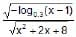is

Detailed Solution for Competition Level Test: Relations And Functions- 1 - Question 1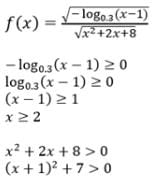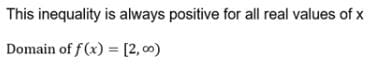Competition Level Test: Relations And Functions- 1 - Question 2

### The domain of the function f(x) = log1/2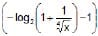is

Competition Level Test: Relations And Functions- 1 - Question 3

### If q2 – 4pr = 0, p > 0, then the domain of the function, f(x) = log (px3 + (p + q) x2 + (q + r) x + r) is

Detailed Solution for Competition Level Test: Relations And Functions- 1 - Question 3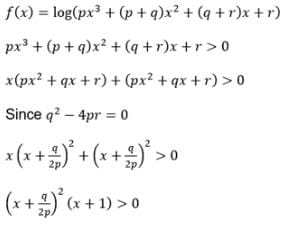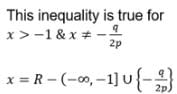Competition Level Test: Relations And Functions- 1 - Question 4

Find domain of the function f(x) =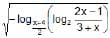Detailed Solution for Competition Level Test: Relations And Functions- 1 - Question 4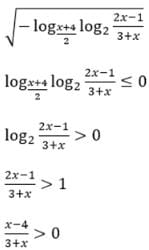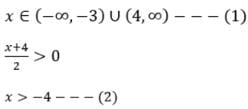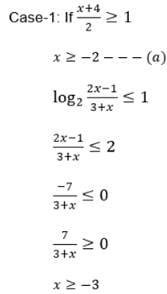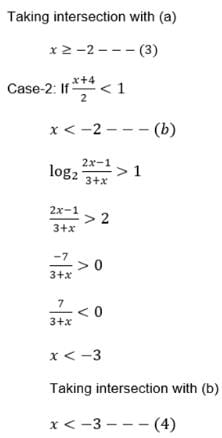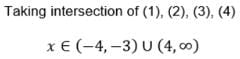Competition Level Test: Relations And Functions- 1 - Question 5

The domain of the function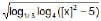is (where [x] denotes greatest integer function)

Detailed Solution for Competition Level Test: Relations And Functions- 1 - Question 5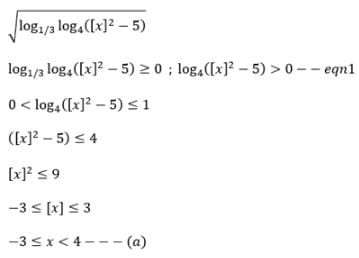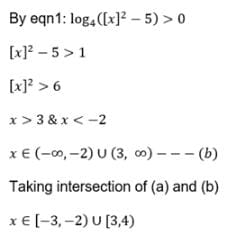Competition Level Test: Relations And Functions- 1 - Question 6

Range of f(x) = 4x + 2x + 1 is

Competition Level Test: Relations And Functions- 1 - Question 7

Range of f(x) = log√5 {√2 (sin x –cos x) + 3} is

Competition Level Test: Relations And Functions- 1 - Question 8

The range of the functin f(x) = log√2(2– log2 (16 sin2 x + 1)) is

Competition Level Test: Relations And Functions- 1 - Question 9

Range of the function f(x)=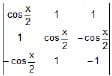is

Detailed Solution for Competition Level Test: Relations And Functions- 1 - Question 9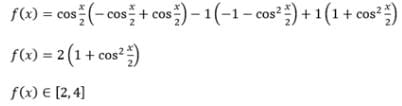Competition Level Test: Relations And Functions- 1 - Question 10

If f(x) =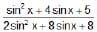, then range of f(x) is

Competition Level Test: Relations And Functions- 1 - Question 11

The sum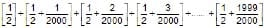is equal to (where [*] denotes the greatest integer function)

Detailed Solution for Competition Level Test: Relations And Functions- 1 - Question 11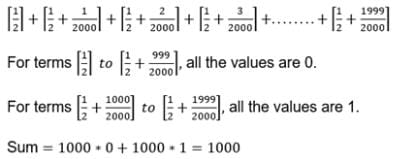Competition Level Test: Relations And Functions- 1 - Question 12

Which of the following represents the graph of f(x) = sgn ([x + 1])

Competition Level Test: Relations And Functions- 1 - Question 13

If f(x) = 2 sin2q+4 cos (x+q) sin x. sin q+cos (2x+2q) then value of f2(x) + f2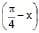is

Competition Level Test: Relations And Functions- 1 - Question 14

Let f(x) = ax2 + bx + c, where a, b, c are rational and f : Z → Z, where Z is the set of integers. Then a+ b is

Detailed Solution for Competition Level Test: Relations And Functions- 1 - Question 14

f : Z → Z
f(x) = ax2 + bx + c
x=0, f(0) = a(0)2 + b(0) + c
= c [it is an integer]
x=1, f(1) = a + b+ c should be an integer
a + b+ c = 1
a + b = 1-c
a+b should be an integer.

Competition Level Test: Relations And Functions- 1 - Question 15

Which one of the following pair of functions are identical ?

Competition Level Test: Relations And Functions- 1 - Question 16

The function f : [2, ∞) → Y defined by f(x) = x2 – 4x + 5 is both one–one & onto if

Competition Level Test: Relations And Functions- 1 - Question 17

Let f : R → R be a function defined by f(x) =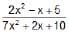then f is

Competition Level Test: Relations And Functions- 1 - Question 18

Let f : R → R be a function defined by f(x) = x3 + x2 + 3x + sin x. Then f is

Competition Level Test: Relations And Functions- 1 - Question 19

If f(x) =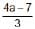x3 + (a – 3) x2 + x + 5 is a one–one function, then

Competition Level Test: Relations And Functions- 1 - Question 20

The graph of the function y = f(x) is symmetrical about the line x = 2, then-

Competition Level Test: Relations And Functions- 1 - Question 21

The function f : R → R defined by f(x) = 6x + 6|x| is

Competition Level Test: Relations And Functions- 1 - Question 22

Let `f' be a function from R to R given by f(x) =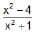. Then f(x) is

Competition Level Test: Relations And Functions- 1 - Question 23

If f(x) = cot-1 x : R+ →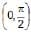and g(x) = 2x – x2 : R → R. Then the range of the function f(g(x)) wherever define is

Detailed Solution for Competition Level Test: Relations And Functions- 1 - Question 23

g(x) = 2x-x2
x(2-x)
-b/2a =. -2/(-2)
=> 1
x implies (0,2)
g(x) implies (0,1]
f(g(x)) = [π/4, π/2)

Competition Level Test: Relations And Functions- 1 - Question 24

Let g(x) = 1 + x – [x] and f(x) =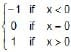, then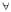x, fog(x) equals (where [*] represents greatest integer function).

Competition Level Test: Relations And Functions- 1 - Question 25

Let f: [0, 1] → [1, 2] defined as f(x) = 1 + x and g : [1, 2] → [0, 1] defined as g(x) = 2 – x then the composite function gof is

Competition Level Test: Relations And Functions- 1 - Question 26

Let f & g be two functions both being defined from R → R as follows f(x) =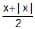and g(x) =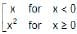. Then

Detailed Solution for Competition Level Test: Relations And Functions- 1 - Question 26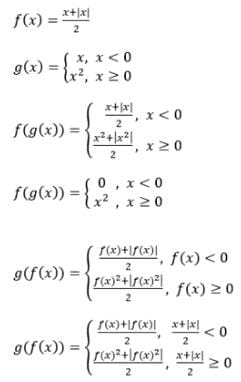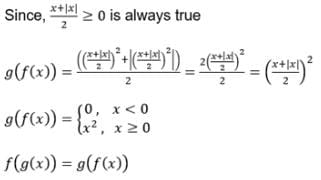Competition Level Test: Relations And Functions- 1 - Question 27

If y = f (x) satisfies the condition f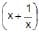= x2 +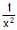(x > 0) then f(x) equals

Competition Level Test: Relations And Functions- 1 - Question 28

The function f(x) = log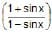is

Competition Level Test: Relations And Functions- 1 - Question 29

It is given that f(x) is an even function and satisfy the relation f(x) =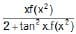then the value of f(10) is

Detailed Solution for Competition Level Test: Relations And Functions- 1 - Question 29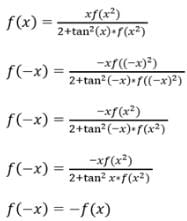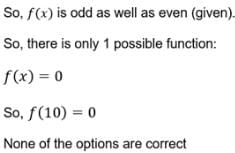Competition Level Test: Relations And Functions- 1 - Question 30

Fundamental period of f(x) = sec (sin x) is

## JEE Foundation

99 videos|291 docs|212 tests
Information about Competition Level Test: Relations And Functions- 1 Page
In this test you can find the Exam questions for Competition Level Test: Relations And Functions- 1 solved & explained in the simplest way possible. Besides giving Questions and answers for Competition Level Test: Relations And Functions- 1, EduRev gives you an ample number of Online tests for practice

## JEE Foundation

99 videos|291 docs|212 tests(Scan QR code)